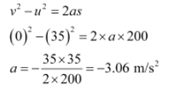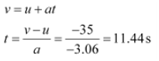## On a two-lane road, car A is travelling with a speed of 36 km h-1. Two cars B and C approach car A in opposite directions with a speed of 54 km h-1 each. At a certain instant, when the distance AB is equal to AC, both being 1 km, B decides to overtake A before C does. What minimum acceleration of car B is required to avoid an accident?

Velocity of car A, vA = 36 km/h = 10 m/s
Velocity of car B, vB = 54 km/h = 15 m/s
Velocity of car C, vC = 54 km/h = 15 m/s
Relative velocity of car B with respect to car A, vBA = vB - vA = 15 - 10 = 5 m/s
Relative velocity of car C with respect to car A, vCA = vC - (- vA) = 15 + 10 = 25 m/s
At a certain instance, both cars B and C are at the same distance from car A i.e., s = 1 km = 1000 m
Time taken (t) by car C to cover 1000 m =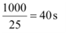Hence, to avoid an accident, car B must cover the same distance in a maximum of 40 s.
From second equation of motion, minimum acceleration (a) produced by car B can be obtained as: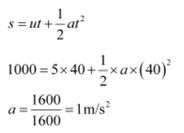## Two trains A and B of length 400 m each are moving on two parallel tracks with a uniform speed of 72 km h-1 in the same direction, with A ahead of B. The driver of B decides to overtake A and accelerates by 1 m/s2. If after 50 s, the guard of B just brushes past the driver of A, what was the original distance between them? Answer:

For train A:
Initial velocity, u = 72 km/h = 20 m/s
Time, t = 50 s
Acceleration, aI = 0 (Since it is moving with a uniform velocity)
From second equation of motion, distance (sI) covered by train A can be obtained as: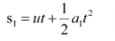= 20 × 50 + 0 = 1000 m
For train B:
Initial velocity, u = 72 km/h = 20 m/s
Acceleration, a = 1 m/s2
Time, t = 50 s
From second equation of motion, distance (sII)covered by train A can be obtained
as: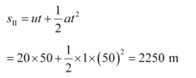Hence, the original distance between the driver of train A and the guard of train B is 2250 -1000 = 1250 m.

## A car moving along a straight highway with a speed of 126 km h-1 is brought to a stop within a distance of 200 m. What is the retardation of the car (assumed uniform), and how long does it take for the car to stop?